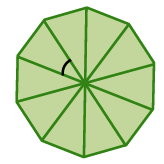### Home > CCG > Chapter 11 > Lesson 11.2.2 > Problem11-99

11-99.

Find the area of a regular decagon if the length of each side is $20$ units.

Find the measure of a central angle.

Use this to determine the height of one of the triangles.

Find the area of one of the triangles.

Find the total area.

$3077.7\text{ units}^2$